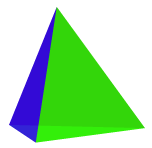# Triangular Pyramid

Go to Surface Area or Volume.

Triangular Pyramid Facts

Notice these interesting things:

• It has 4 Faces
• The 3 Side Faces are Triangles
• The Base is also a Triangle
• It has 4 Vertices (corner points)
• It has 6 Edges
• It is also a Tetrahedron

## Surface AreaWhen side faces are the same:

Surface Area = [Base Area] + 1 2 × Perimeter × [Slant Length]

### Example: Base Area is 28, Perimeter is 20, Slant length is 5

Surface Area = [Base Area] + 1 2 × Perimeter × [Slant Length]
= 28 + 1 2 × 20 × 5
= 28 + 50
= 78

When side faces are different we can calculate the area of the base and each triangular face separately and then add them up.

## Volume

Volume = 1 3 × [Base Area] × Height

### Example: base area is 28, height is 4.5

Volume= 1 3 × [Base Area] × Height
= 1 3 × 28 × 4.5
= 1 3 × 126
= 42